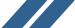Multivariate Calculus Study Guide - Calculus Software, Calculus Homework, Calculus Help - Maplesoft
Included in Maple!

# Multivariate Calculus Study Guide

Succeed in your Multivariate Calculus course with this Maple e-book.

##Why choose the Clickable Multivariate Calculus Study Guide?• An interactive e-book designed to help you succeed in your Multivariate Calculus course.
• You’ll never again think you’ve misunderstood a concept when really you just lost a minus sign. While you are learning, you can leave the mechanics of the computations to Maple so you can focus on understanding the new ideas.
• The many plots and animations help you actually see what is going on in your problem and what your solution means.
• With over 700 worked problems taken from more than 50 topics from multivariate calculus, you will always find the help you need. This guide completely covers all topics from a standard multivariate calculus course.
• You’ll learn how to easily visualize and solve your own multivariate calculus problems in Maple, so you can check your homework and get extra practice.
• The perfect companion product to Maple - Student EditionWhat makes it "Clickable"?

This study guide makes extensive use of Maple’s Clickable Math approach. Almost all the examples in this guide are solved using interactive point-and-click techniques – no commands or syntax required. This means you can let Maple take care of the details while you stay focused on the math. You can easily apply these same Clickable Math techniques to solving other math problems, whether they are from calculus or your other technical courses.

And for those who prefer to use commands, command-based solutions are also provided for almost every example in this book. The choice is yours!

## Topics

#### Vectors, Lines and Planes

• Cartesian Coordinates and Vectors
• Vector Arithmetic
• Dot Product
• Cross Product
• Applications of Vector Products
• Lines
• Planes

#### Space Curves

• Position-Vector Representation
• Arc Length as Parameter
• Tangent Vectors
• Curvature
• Principal Normal
• Binormal and Torsion
• Frenet-Serret Formalism
• Resolution of R" along T and N
• Applications to Dynamics

#### Functions of Several Variables

• Functions and Their Graphs
• Limits and Continuity

#### Partial Differentiation

• First-Order Partial Derivatives
• Higher-Order Partial Derivatives
• Chain Rule
• Directional Derivative
• Surface Normal and Tangent Plane
• Approximations
• Unconstrained Optimization
• Constrained Optimization
• Optimization on Closed Domains
• Differentiability

#### Double Integration

• The Double Integral
• Iterated Double Integrals
• Regions with Curved Boundaries
• Changing the Order of Iteration
• Numeric Evaluation of Iterated Integrals
• Changing Variables in a Double Integral
• Double Integration in Polar Coordinates

#### Applications of Double Integration

• Area
• Volume
• Surface Area
• Average Value
• First Moments
• Second Moments

#### Triple Integration

• The Triple Integral
• Iterated Triple Integrals
• Regions with Curved Boundaries
• Integration in Cylindrical Coordinates
• Spherical Coordinates
• Integration in Spherical Coordinates

#### Applications of Triple Integration

• Volume
• Average Value
• First Moments
• Moments of Inertia (Second Moments)
• Changing Variables in a Triple Integral

#### Vector Calculus New!

• Vector Objects
• Differential Operators
• Differential Identities
• Line Integrals
• Surface Integrals
• Conservative and Solenoidal Fields
• Divergence Theorem
• Stokes’ Theorem
• Green’s Theorem
Recorded Webinar: Reviewing the Multivariate Calculus Study Guide
This webinar explores the Multivariate Calculus Study Guide, showing how it uses all the best tools Maple has available for mastering the material of the multivariate calculus course.